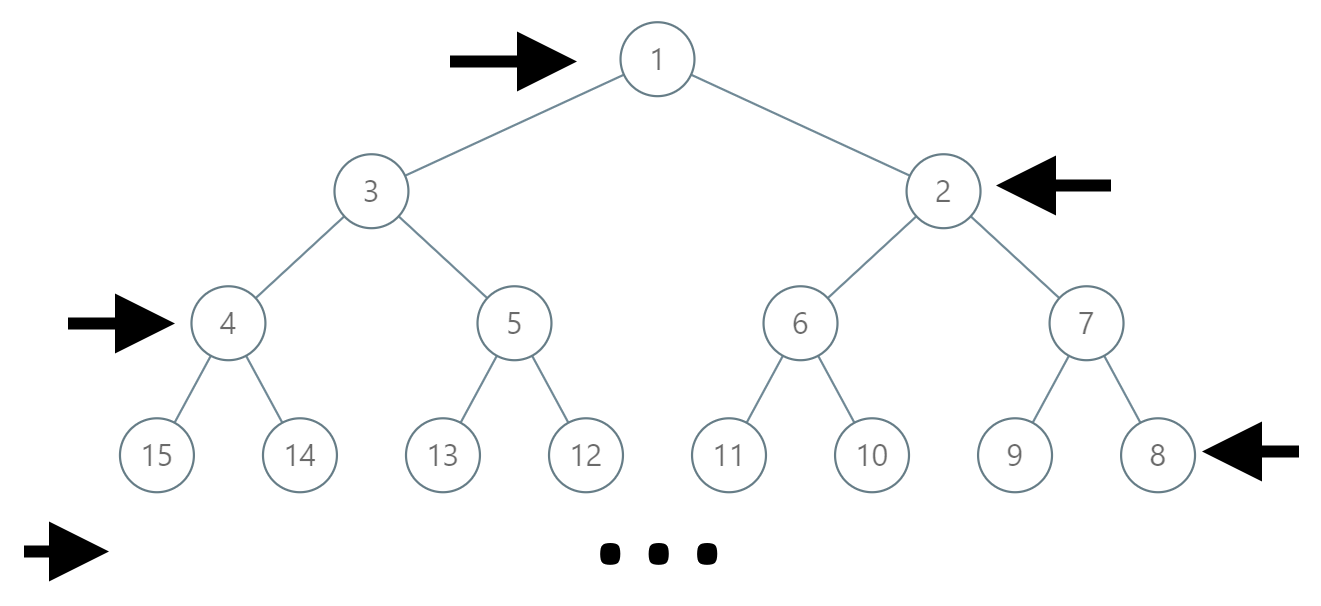1104. Path In Zigzag Labelled Binary Tree

In an infinite binary tree where every node has two children, the nodes are labelled in row order.

In the odd numbered rows (ie., the first, third, fifth,...), the labelling is left to right, while in the even numbered rows (second, fourth, sixth,...), the labelling is right to left.Given the `label` of a node in this tree, return the labels in the path from the root of the tree to the node with that `label`.

Example 1:

```Input: label = 14
Output: [1,3,4,14]
```

Example 2:

```Input: label = 26
Output: [1,2,6,10,26]
```

Constraints:

• `1 <= label <= 10^6`

1104. Path In Zigzag Labelled Binary Tree
``````struct Solution;

impl Solution {
fn path_in_zig_zag_tree(mut label: i32) -> Vec<i32> {
let mut level = 0;
while label >= 1 << level {
level += 1;
}
let mut res = vec![0; level];
for i in (0..level).rev() {
res[i] = label;
let r = (1 << i) - 1;
let l = r / 2 + 1;
label = l + r - label / 2;
}
res
}
}

#[test]
fn test() {
let label = 14;
let res = vec![1, 3, 4, 14];
assert_eq!(Solution::path_in_zig_zag_tree(label), res);
let label = 26;
let res = vec![1, 2, 6, 10, 26];
assert_eq!(Solution::path_in_zig_zag_tree(label), res);
let label = 16;
let res = vec![1, 3, 4, 15, 16];
assert_eq!(Solution::path_in_zig_zag_tree(label), res);
}
``````